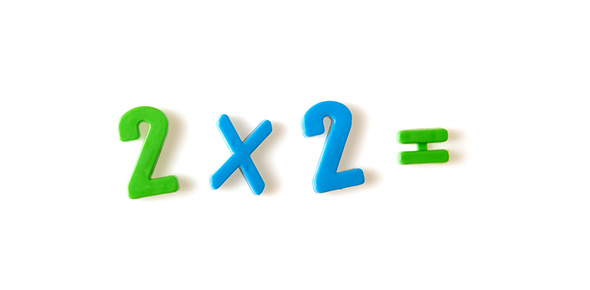# Multiplication Properties

12 Questions | Total Attempts: 1093SettingsRelated Topics
• 1.
9 x 3 = 3 x 9
• A.

Associative Property

• B.

Commutative Property

• C.

Multiplicative Property

• D.

Distributive Property

• 2.
61 x 1 = 61
• A.

Associative Property

• B.

Commutative Property

• C.

Multiplicative Property

• D.

Distributive Property

• 3.
11 x (5 x 6) = (11 x 5) x 6
• A.

Distributive Property

• B.

Multiplicative Property

• C.

Associative Property

• D.

Commutative Property

• 4.
58 x 27 = 27 x 58
• A.

Distributive Property

• B.

Multiplicative Property

• C.

Associative Property

• D.

Commutative Property

• 5.
3 x (5 + 8) = (3 x 5) + (3 x 8)
• A.

Commutative Property

• B.

Associative Property

• C.

Distributive Property

• D.

Multiplicative Property

• 6.
Which equation shows the commutative property of multiplication?
• A.

0 x 3 - 0 x 2 = 0 x (3 - 2)

• B.

4 x 7 = 7 x 4

• C.

0 = 0 x 5

• D.

9 x 2 = 9 + 9

• 7.
Which property of multiplication is shown in 5 = 1 x 5?
• A.

Multiplicative Identity Property

• B.

Zero Property

• C.

Distributive Property

• D.

Associative Property

• 8.
Which equation shows the zero property of multiplication?
• A.

3 x (6 + 1) = (3 x 6) + (3 x 1)

• B.

3 x 7 - 2 x 7 = (3 - 2) x 7

• C.

0 = 0 x 9

• D.

(4 + 1) x 8 = 4 x 8 + 1 x 8

• 9.
Which equation shows the associative property of multiplication?
• A.

(4 x 9) x 2 = 4 x (9 x 2)

• B.

1 x (3 + 0) = 1 x 3 + 3 x 0

• C.

6 = 1 x 6

• D.

2 = 2 x 1

• 10.
Which shows the distributive property of multiplication?
• A.

7 x 8 = 8 x 7

• B.

(3 x 8) x 4 = 3 x (8 x 4)

• C.

6 = 6 x 1

• D.

(6 + 9) x 7 = (6 x7) + (9 x 7)

• 11.
Which property of multiplication is shown?2 x 3 = 3 x 2
• A.

Identity

• B.

Commutatitve

• C.

Associative

• D.

Zero

• 12.
Which property of multiplication is shown?8 = 1 x 8
• A.

Distributive

• B.

Associative

• C.

Identity

• D.

Zero